# [Problem Set 2 Quantitative models of behavior] Problem 1: Rescola-Wagner Rule

Link of the iPython notebook for the code

# PROBLEM 1 - Rescorla-Wagner Model

Let us model a classical conditioning experiment with resort to the Rescorla-Wagner rule.

The experiment is as follows: at trial $i ∈ ℕ$:

• an animal may or may not be presented with a stimulus $u_i$ (e.g.: ringing a bell, clapping hands): $u_i = 1$ if the stimulus is present, $u_i = 0$ otherwise
• … which may or may not in turn be followed by a reward $r_i$ (e.g.: food): $r_i = 1$ if there is a reward, $r_i = 0$ otherwise

The animal is assumed to be willing to predict the correlation between the stimulus and the reward (e.g: by salivating in anticipation): i.e. to predict if it will get the reward based on the presence or absence of the stimulus. As such, the prediction of the animal is denoted by $v_i$ at trial $i$:

$∀i, \; v_i ≝ w_i u_i$

where $w$ is a parameter learned and updated - trial after trial - by the animal, in accordance with the following Rescola-Wagner rule:

$w_i → w_i + \underbrace{ε}_{\text{learning rate } << 1} \underbrace{(r_i − v_i)}_{≝ \, δ_i} u_i$

## 1. Introductory example

For instance, let us suppose that there are $50$ trials, and:

• during the first $25$ trials, both stimuli and reward are present ($u_i = r_i = 1$)
• during the last $25$ ones, only the stimulus is present ($u_i = 1, \, r_i = 0$)

as illustrated by the following figure: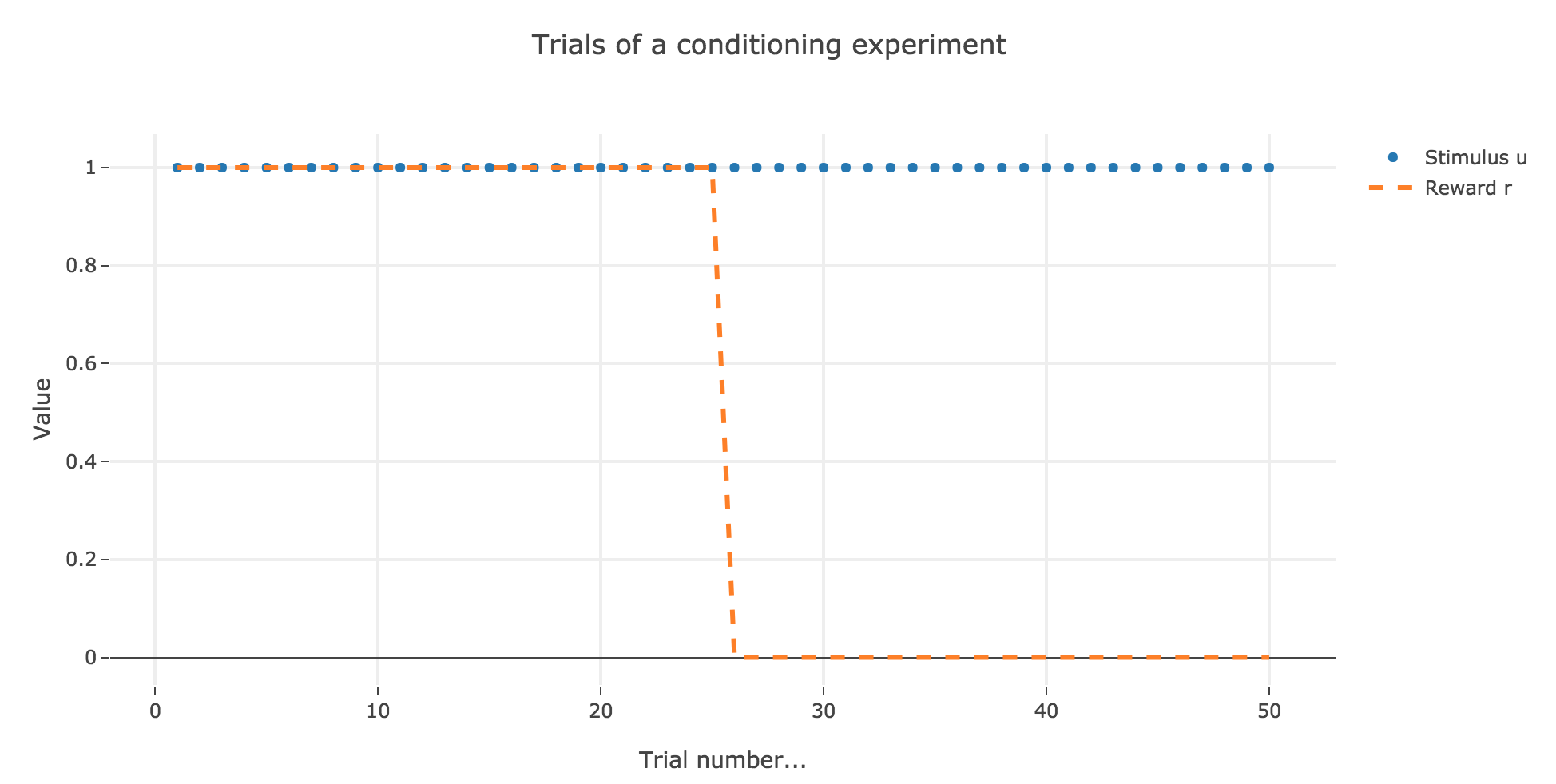Figure 1. - Introductory example: plot of stimulus and reward as a function of the trial index

In which case, according to the Rescola-Wagner rule, the animal prediction $v_i$ is as follows: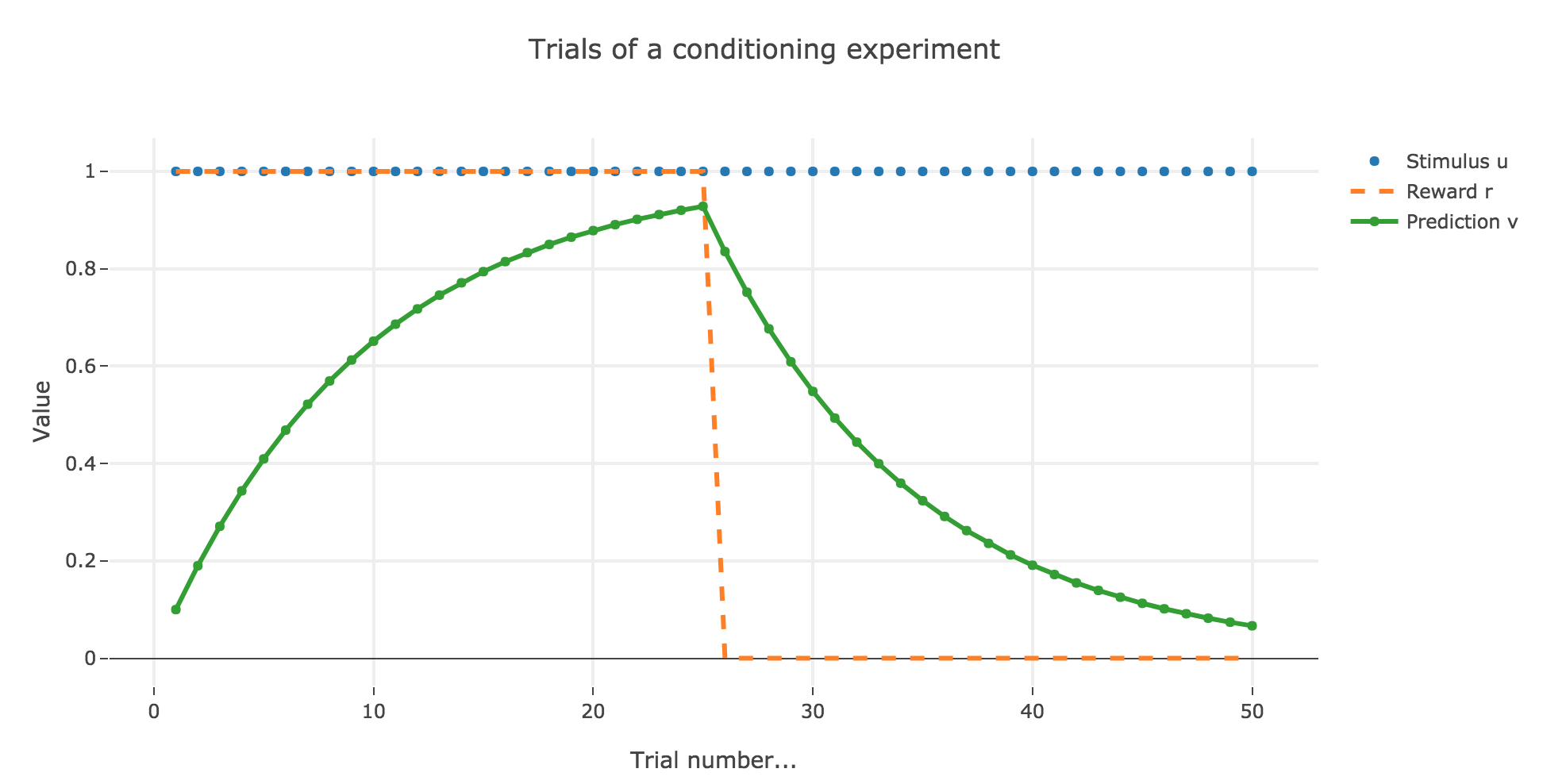Figure 1.2. - Introductory example: plot of the prediction $v$, with $ε = 0.1$ as a function of the trial index

where the learning rate $ε$ is set to be $0.1$.

It appears that the animal prediction $v = wu$ does tend toward the reward. Indeed, if $r_i ≝ r_0$ and $u_i ≝ u_0$ are constant (as it happens here for the first $25$ trials, and for the last $25$ ones):

\begin{align*} w_{i+1} & = w_i + ε (r_0 − u_0 w_i) u_0 \\ & = (1- ε u_0^2) w_i + ε r_0 u_0 \\ \end{align*}

Therefore:

\begin{align*} ∀i ≥ i_0, \; w_i &= (1- ε u_0^2)^{i-i_0} \Big(w_{i_0} - \frac{ε r_0 u_0}{1-(1-ε u_0^2)}\Big) + \frac{ε r_0 u_0}{1-(1-ε u_0^2)}\\ &= \frac{r_0}{u_0}\Big(1 - (1-ε u_0^2)^{i-i_0}\Big) + w_{i_0} (1-ε u_0^2)^{i-i_0} \\ \end{align*}

As a result:

• for the first $25$ trials: $r_0 = u_0 = 1$ and $w_0 = i_0 = 0$, so that:

$∀ i ∈ \lbrace 0, 24 \rbrace, \; w_i = 1 - (1-ε)^i \xrightarrow[i \to +∞]{} 1 = r_0$
• for the last $25$ trials: $r_0 = 0, \; u_0 = 1$ and $i_0 = 24, \, w_{24} > 0$, so that:

$∀ i ∈ \lbrace 25, 50 \rbrace, \; w_i = w_{24} (1-ε)^{i-24} \xrightarrow[i \to +∞]{} 0 = r_0$

### Changing the learning parameter $ε$

Now, let us change the learning rate $ε$ in the previous example.

#### For $ε ≤ 1$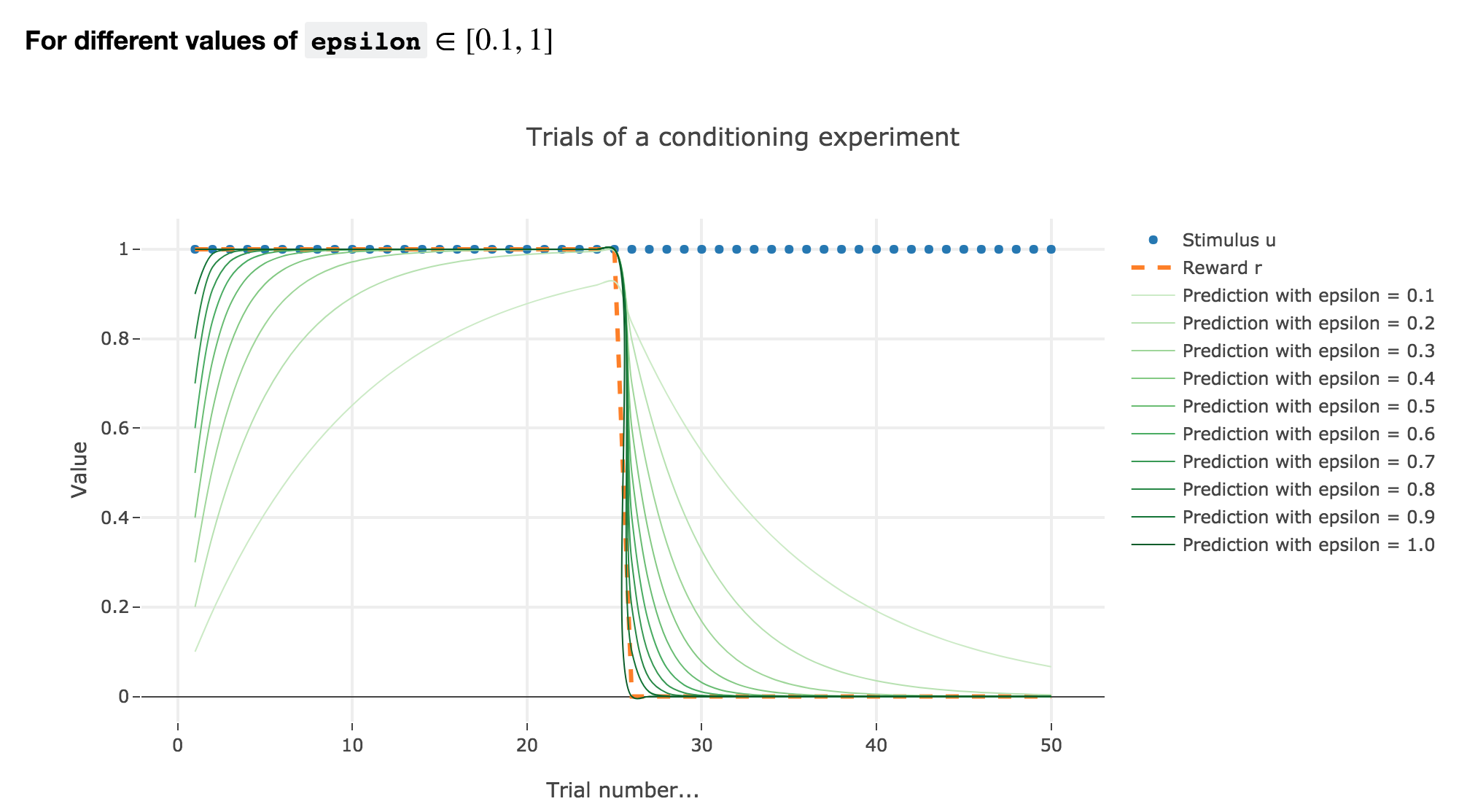Figure 1.3. - Introductory example: plot of the prediction $v$ as a function of the trial index for different values of $0.1 ≤ ε ≤ 1$

As illustrated by the figure above, when $ε ≤ 1$: the bigger the learning rate $ε$, the faster the animal prediction converges toward the reward.

Indeed, as shown above:

$\begin{cases} ∀ i ∈ \lbrace 0, 24 \rbrace, \; w_i = 1 - (1-ε)^i \\ ∀ i ∈ \lbrace 25, 50 \rbrace, \; w_i = w_{24} (1-ε)^{i-24} \end{cases}$

so the bigger the learning rate $ε$, the lower the term $γ ≝ (1-ε)$, and the faster

• $w_i = 1 - γ^i$ converges toward the reward $1$ for the first $25$ trials
• $w_i = w_{24} γ^{i-24}$ converges toward the reward $0$ for the last $25$ trials

It can easily be shown that this observation holds for any constant piecewise reward.

#### For $ε ∈ [1, 2[$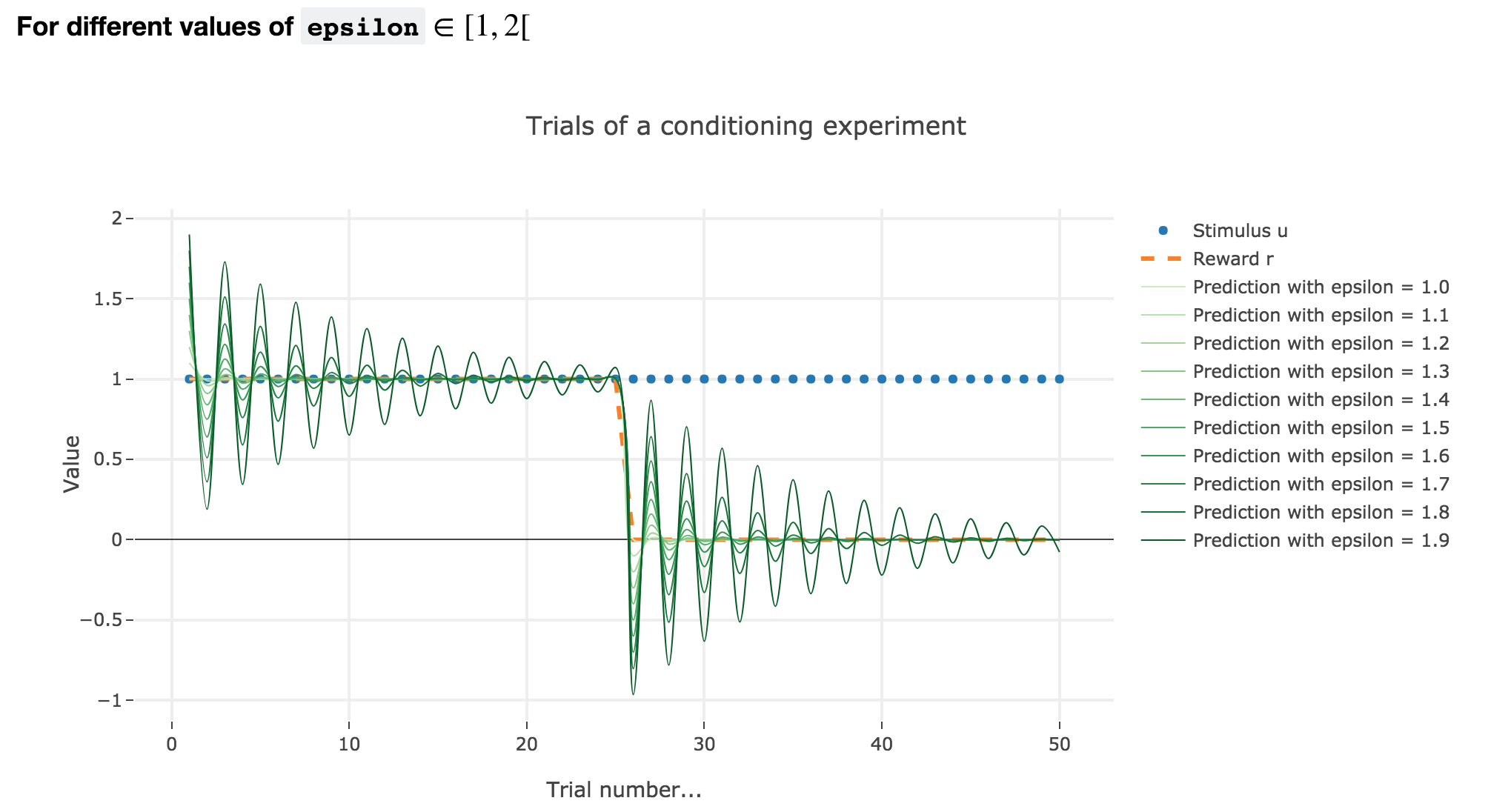Figure 1.4. - Introductory example: plot of the prediction $v$ as a function of the trial index for different values of $1 ≤ ε < 2$

Analogously, if $ε ∈ [1, 2[$: the term $γ ≝ (1-ε) ∈ ]-1, 0]$, and for the first (resp. last) $25$ trials: $w_i = 1 - γ^i$ (resp. $w_i = w_{24} γ^{i-24}$) converges toward the reward $1$ (resp. $0$) while oscillating around it (since $γ ≤ 0$). The oscillation amplitude is all the more significant that $\vert γ \vert$ is big, i.e. that $ε$ is big.

Again, this result can be extended to any constant piecewise reward.

#### For $ε ≥ 2$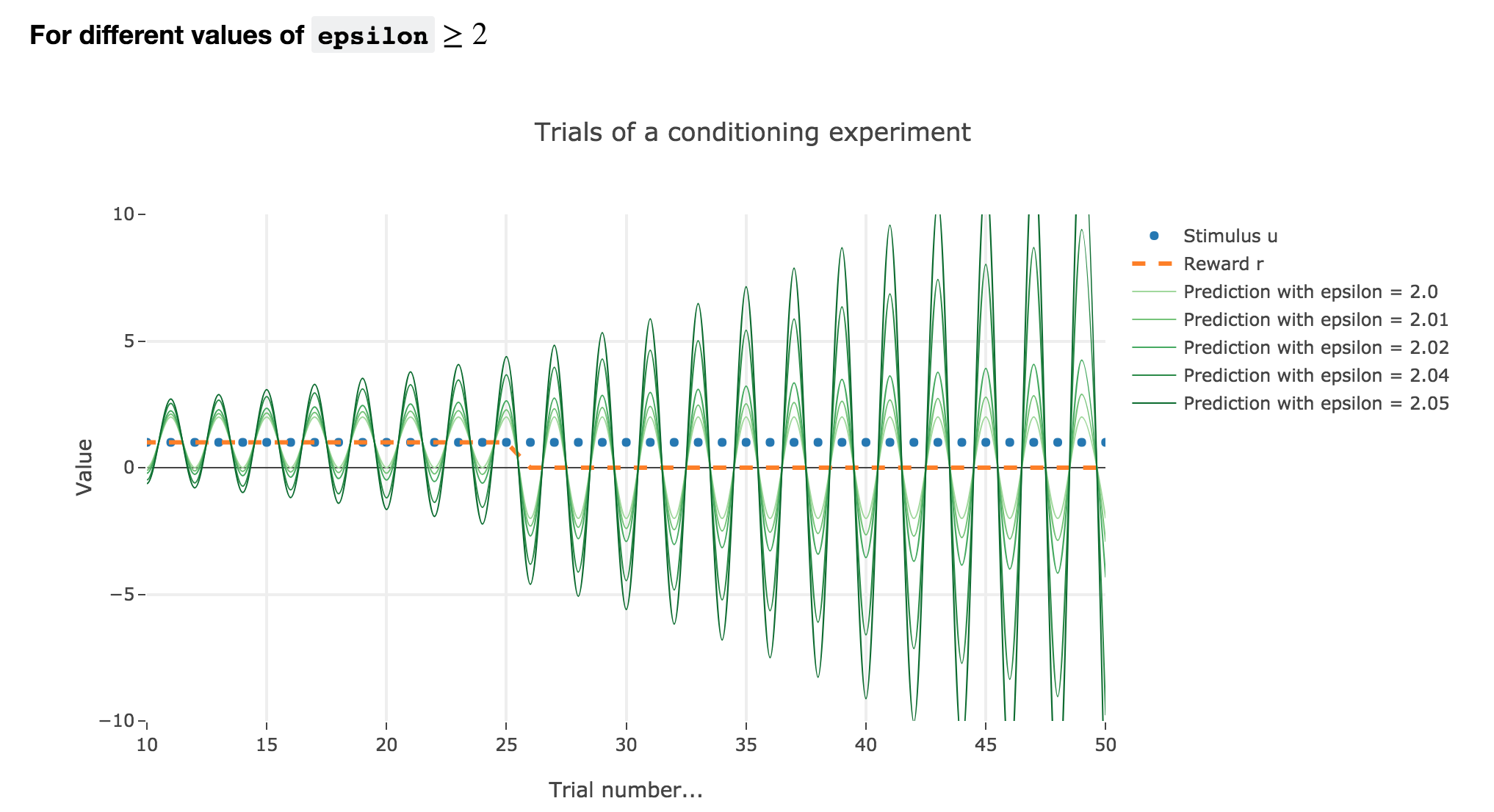Figure 1.5. - Introductory example: plot of the prediction $v$ as a function of the trial index for different values of $ε ≥ 2$

Finally, if $ε ≥ 2$: the term $γ ≝ (1-ε) ≤ -1$, and $w_i$ diverges as $γ^i$ does, while oscillating around it (since $γ ≤ 0$). The oscillation amplitude is all the more significant that $\vert γ \vert$ is big, i.e. that $ε$ is big.

Again, this result stands for any constant piecewise reward.

Going back to our conditioning experiment:

• $ε ≤ 1$ corresponds to what is expected from the experiments: the bigger the learning rate, the faster the convergence of the animal prediction, monotonously for a constant reward: hence the name learning rate.

• $ε ∈ [1 , 2[$ and $ε ≥ 2$ are both degenerate cases: $ε ≥ 2$ is completely nonsensical from a biological standpoint, and the oscillations of $ε ∈ [1 , 2[$ are not what we want to model, which is rather a convergence of the animal prediction straight to the reward.

## 2. Partial conditioning

Let us suppose that in each trial, the stimulus is present, but the presence of the reward is a random event with probability $0.4$.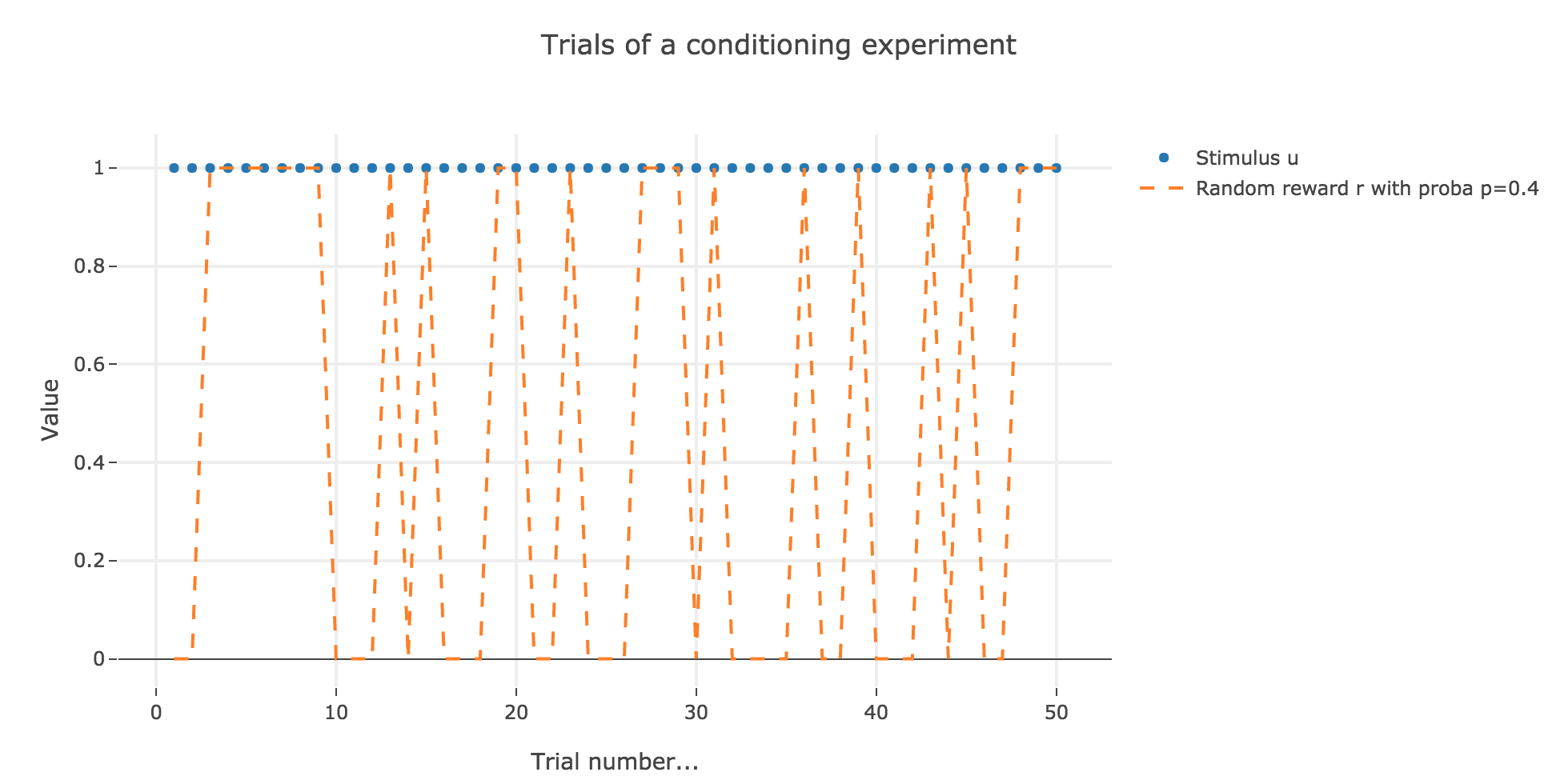Figure 2.1. - Partial conditioning: plot of the reward as a function of the trial index

Similarly to what has been done in the previous section: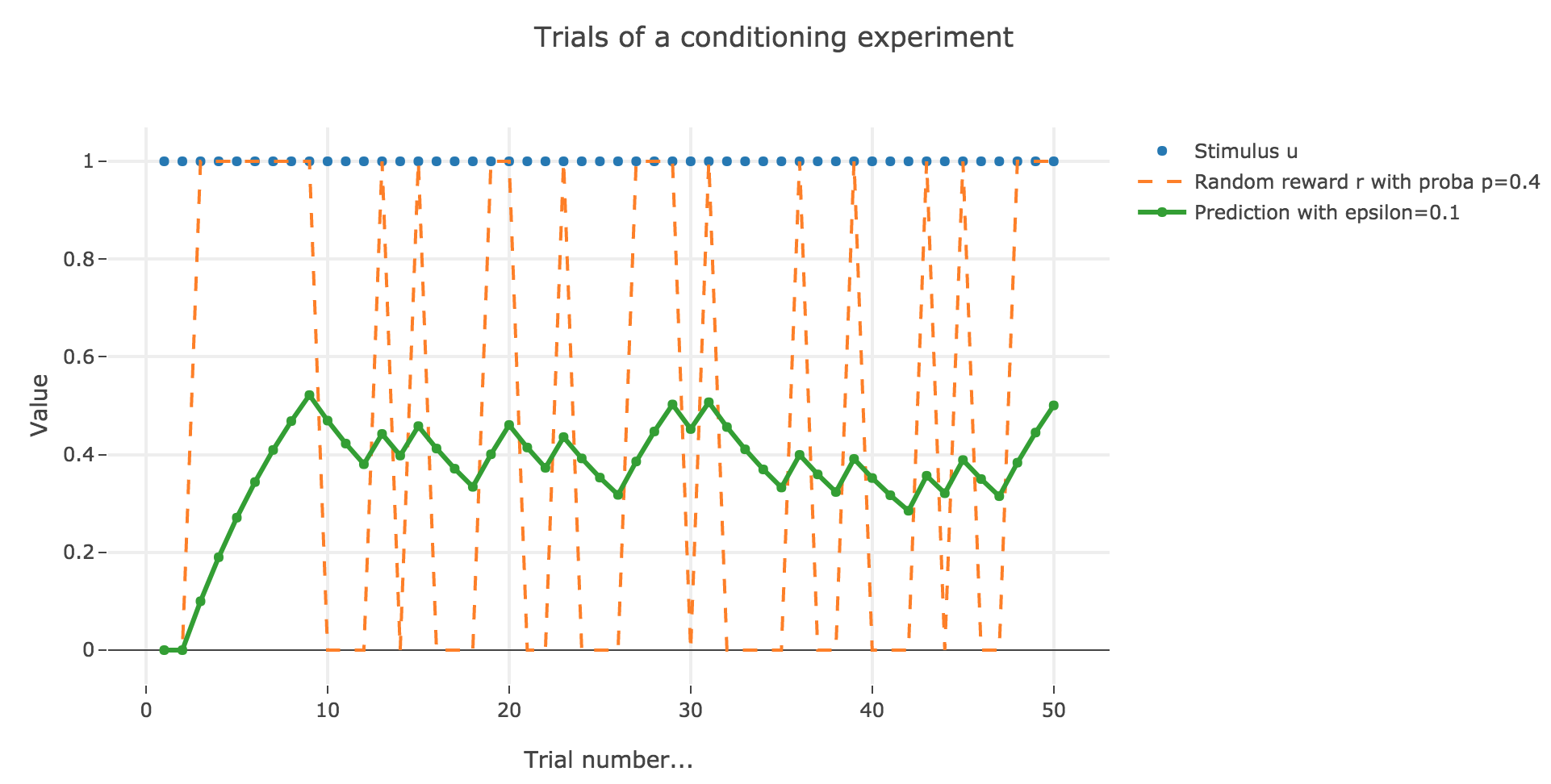Figure 2.2. - Partial conditioning: plot of the animal prediction as a function of the trial index, for $ε = 0.1$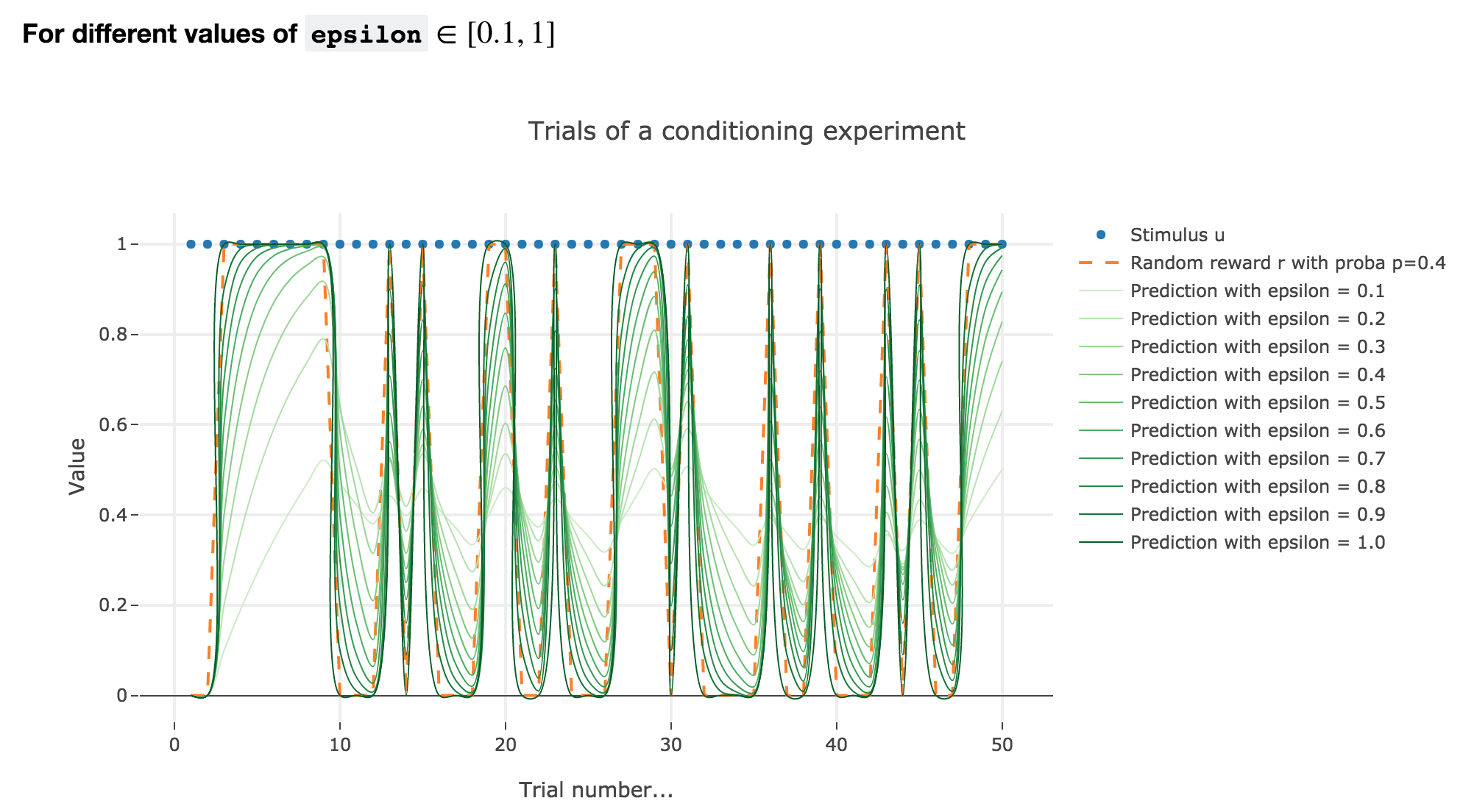Figure 2.3. - Partial conditioning: plot of the animal prediction as a function of the trial index for different values of $ε ∈ [0.1, 1]$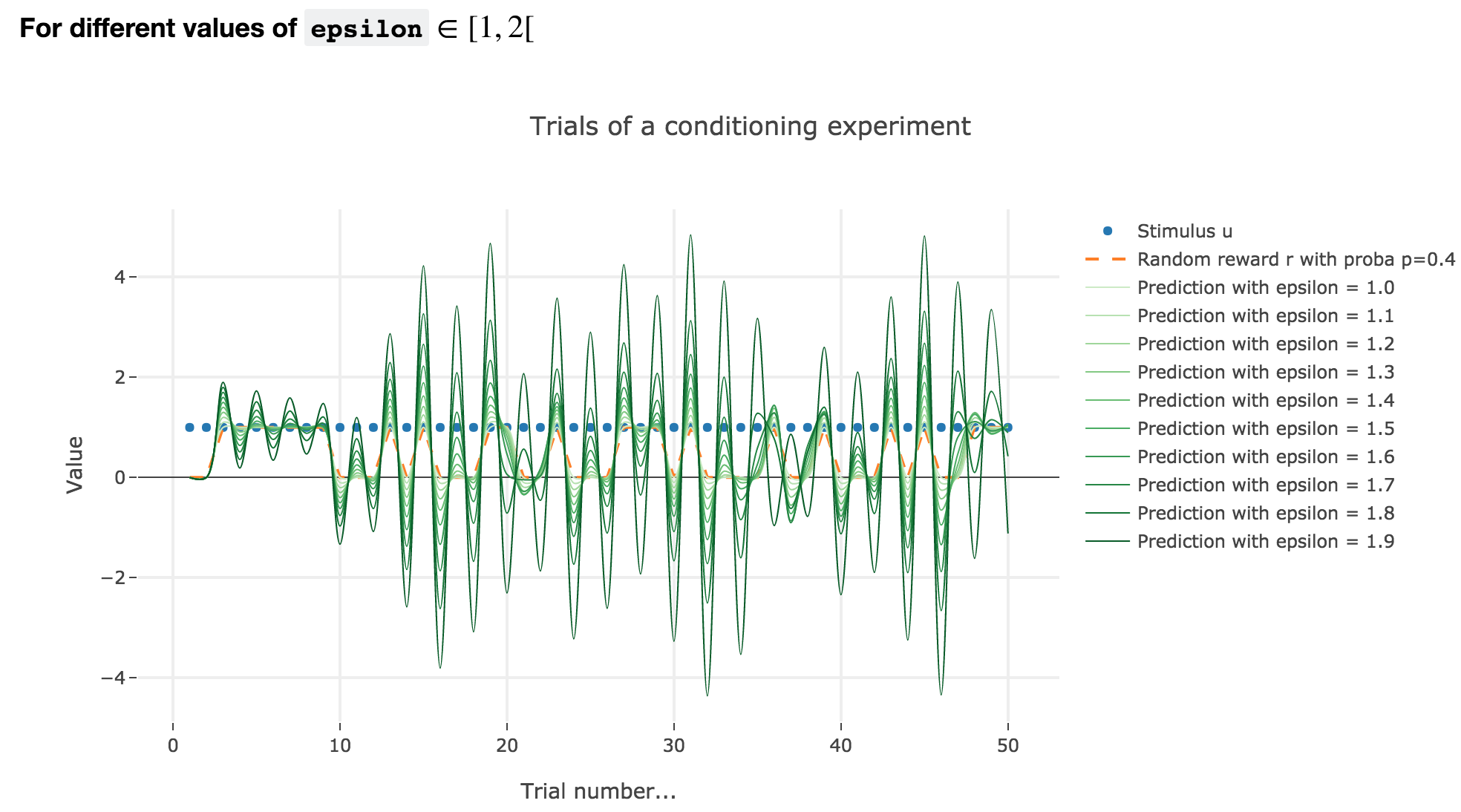Figure 2.4. - Partial conditioning: plot of the animal prediction as a function of the trial index for different values of $ε ∈ [1, 2[$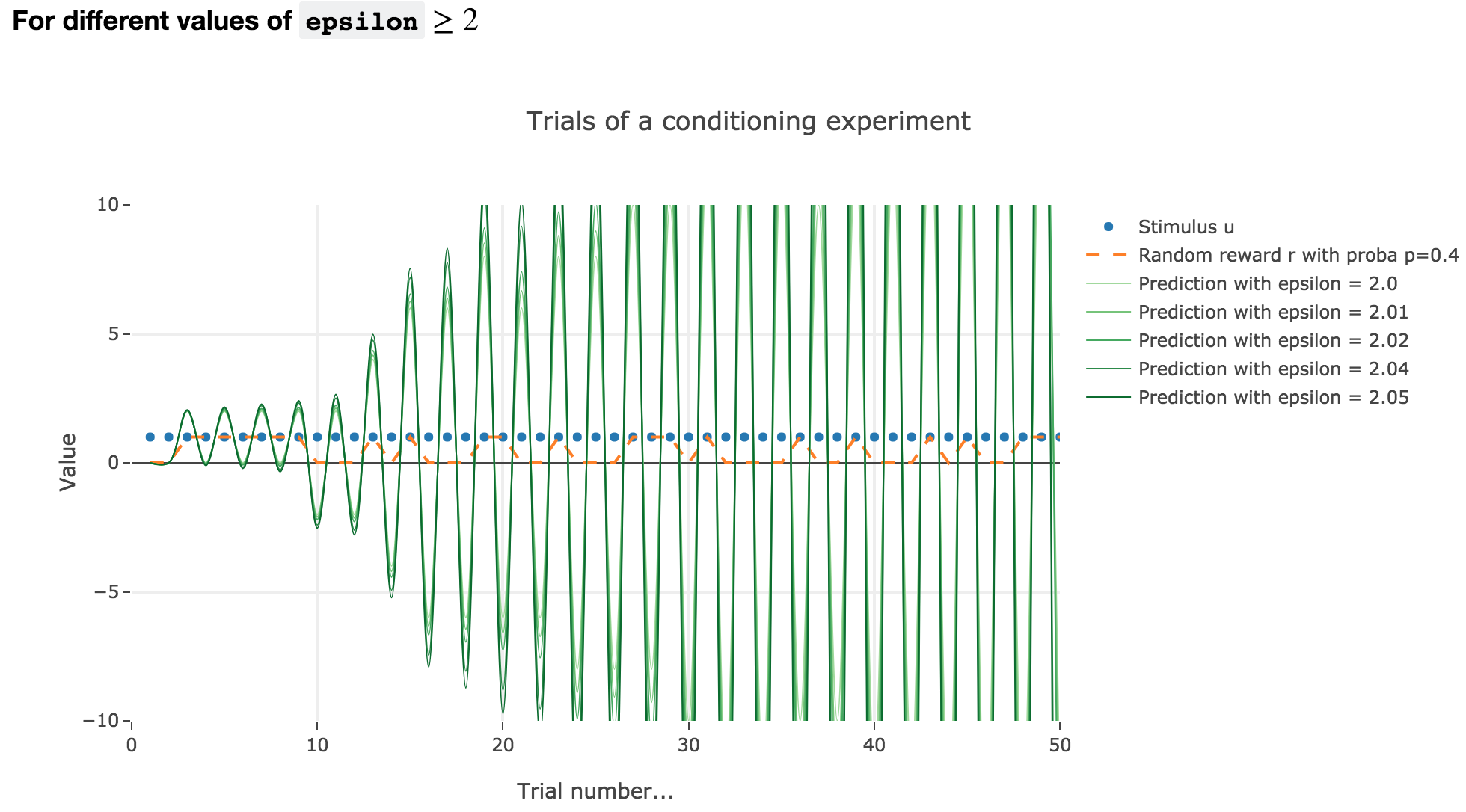Figure 2.5. - Partial conditioning: plot of the animal prediction as a function of the trial index for different values of $ε ≥ 2$

As it happens, the reward is a constant piecewise function, so the previous analysis applies here, by focusing on each segment where the reward is constant (the stimulus is always equal to $1$ here, which simplifies things up). On top of that, we may stress that the shorter these segments are, the higher $ε ≤ 1$ has to be, for the animal to learn the reward within these shorter time spans. The oscillating cases are still irrelevant from a biological standpoint.

## 3. Blocking

Now, we assume that there are two stimuli, $u_1$ and $u_2$, and two parameters, $w_1$ and $w_2$ to learn, and that the animal prediction is given by $v = w_1 u_1 + w_2 u_2$.

During the first $25$ trials, only one stimulus and the reward are present, during the next $25$ trials, both stimuli and the reward are present.

For the record, here is what happens when there is one single stimulus and $u = r = 1$: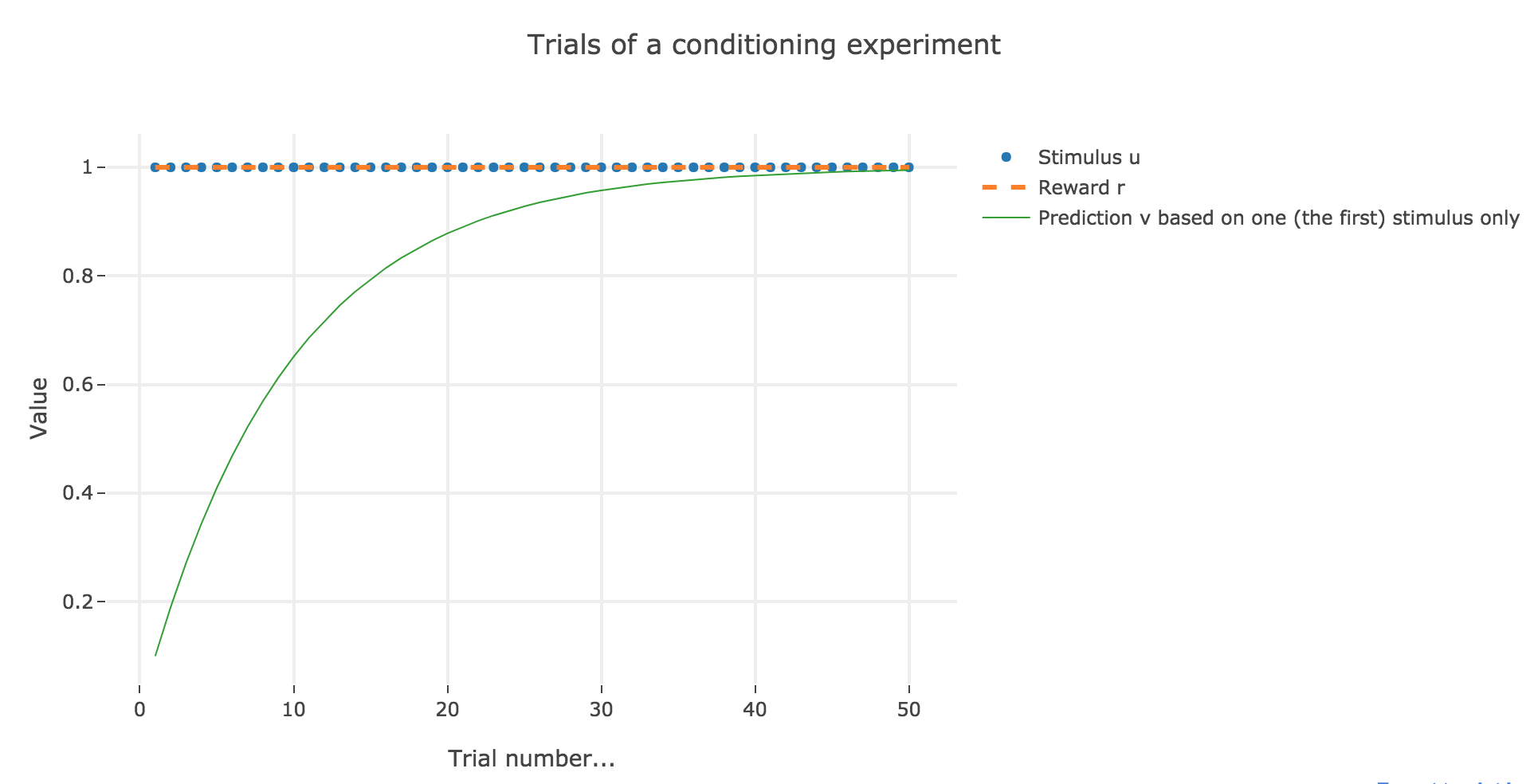Figure 3.1. - Plot of the animal prediction as a function of the trial index for one single stimulus ($ε = 0.1$)

Now, with aforementioned two stimuli: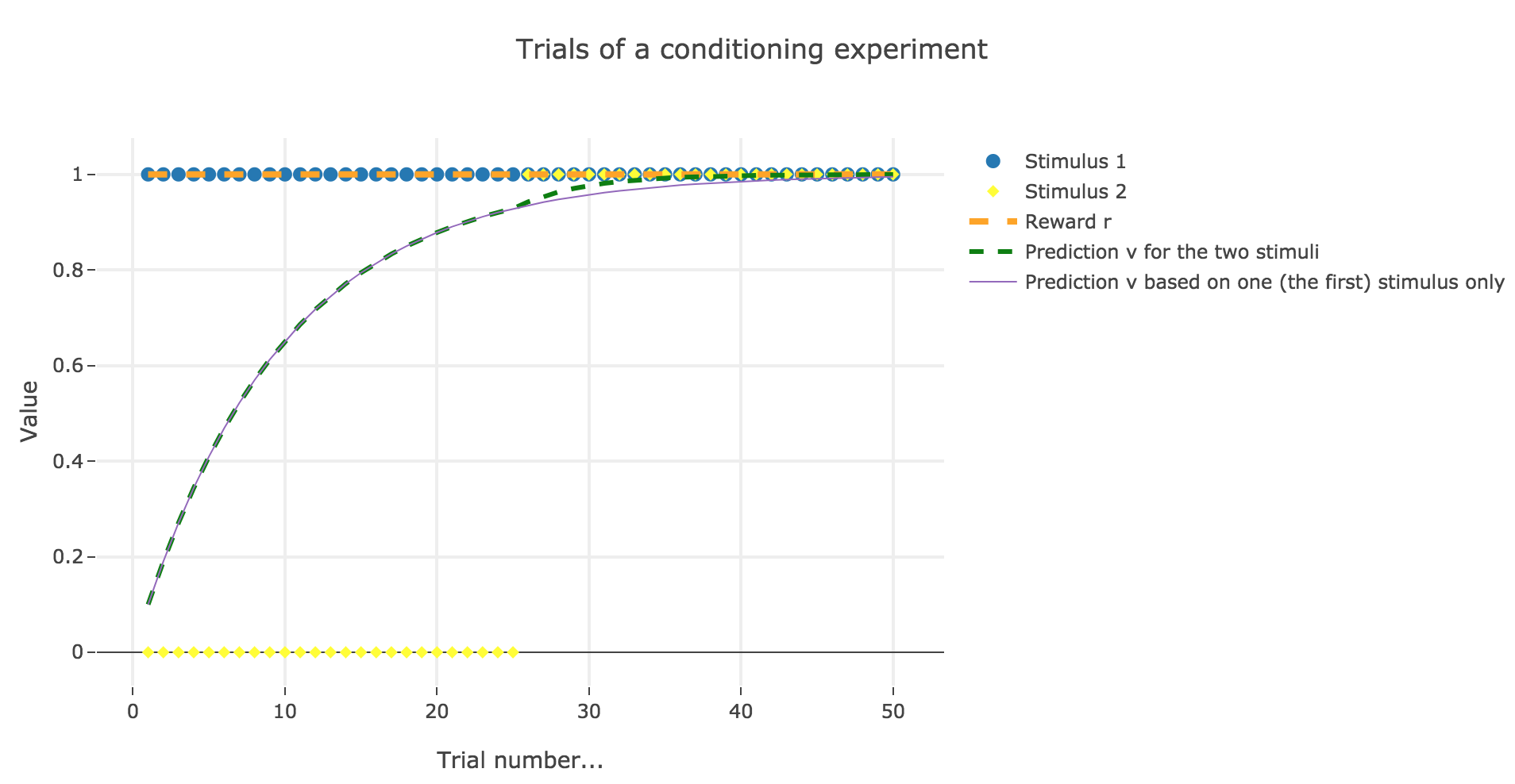Figure 3.2. - Plot of the animal prediction for two stimuli $u_1, u_2$ and one single stimulus $u_1$ ($ε = 0.1$)

To be more precise, here is the evolution of $w_1$ and $w_2$ throughout the trials:

Trial $w_1$ $w_2$
1 0.1 0.
2 0.19 0.
3 0.271 0.
4 0.3439 0.
5 0.40951 0.
6 0.468559 0.
7 0.5217031 0.
8 0.56953279 0.
9 0.61257951 0.
10 0.65132156 0.
11 0.6861894 0.
12 0.71757046 0.
13 0.74581342 0.
14 0.77123208 0.
15 0.79410887 0.
16 0.81469798 0.
17 0.83322818 0.
18 0.84990536 0.
19 0.86491483 0.
20 0.87842335 0.
21 0.89058101 0.
22 0.90152291 0.
23 0.91137062 0.
24 0.92023356 0.
25 0.9282102 0.
26 0.93538918 0.00717898
27 0.94113237 0.01292216
28 0.94572691 0.01751671
29 0.94940255 0.02119235
30 0.95234306 0.02413286
31 0.95469547 0.02648527
32 0.95657739 0.02836719
33 0.95808294 0.02987273
34 0.95928737 0.03107717
35 0.96025092 0.03204071
36 0.96102175 0.03281155
37 0.96163842 0.03342822
38 0.96213176 0.03392156
39 0.96252643 0.03431623
40 0.96284216 0.03463196
41 0.96309475 0.03488455
42 0.96329682 0.03508662
43 0.96345848 0.03524827
44 0.9635878 0.0353776
45 0.96369126 0.03548106
46 0.96377403 0.03556383
47 0.96384024 0.03563004
48 0.96389321 0.03568301
49 0.96393559 0.03572539
50 0.96396949 0.03575929

There is a phenomenon of blocking in the sense that: when $u_2$ appears (from the trial $26$ on), the prediction error is already close to zero (the animal has already almost exclusively associated the presence of the reward with the presence of the first stimulus only), so that the second stimulus is hardly taken into account, compared to the first one (hence the blocking).

We still suppose that there are two stimuli and two parameters to learn. However, now:

• both stimuli and the reward are present throughout all the trials.

• one of the learning rates is larger: $ε_1 = 0.2$ for the first stimulus, and $ε_2 = 0.1$ for the other one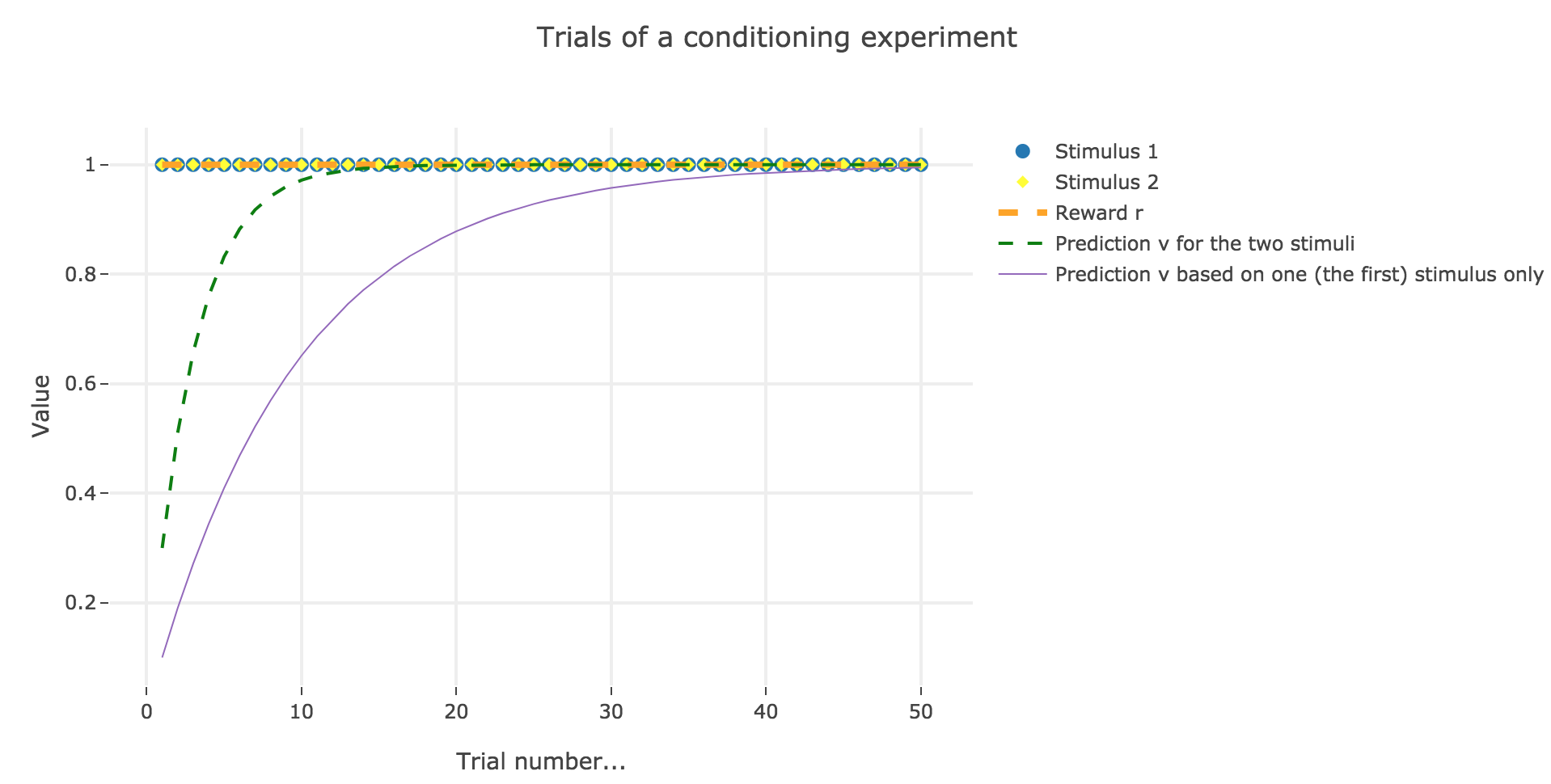Figure 4.1. - Plot of the animal prediction for two stimuli $u_1, u_2$ (with $ε_1 = 0.2, ε_2 = 0.1$) and one single stimulus $u_1$ (with $ε = 0.1$)

Here is the evolution of $w_1$ and $w_2$ throughout the trials:

Trial $w_1$ $w_2$
1 0.2 0.1
2 0.34 0.17
3 0.438 0.219
4 0.5066 0.2533
5 0.55462 0.27731
6 0.588234 0.294117
7 0.6117638 0.3058819
8 0.62823466 0.31411733
9 0.63976426 0.31988213
10 0.64783498 0.32391749
11 0.65348449 0.32674224
12 0.65743914 0.32871957
13 0.6602074 0.3301037
14 0.66214518 0.33107259
15 0.66350163 0.33175081
16 0.66445114 0.33222557
17 0.6651158 0.3325579
18 0.66558106 0.33279053
19 0.66590674 0.33295337
20 0.66613472 0.33306736
21 0.6662943 0.33314715
22 0.66640601 0.33320301
23 0.66648421 0.3332421
24 0.66653895 0.33326947
25 0.66657726 0.33328863
26 0.66660408 0.33330204
27 0.66662286 0.33331143
28 0.666636 0.333318
29 0.6666452 0.3333226
30 0.66665164 0.33332582
31 0.66665615 0.33332807
32 0.6666593 0.33332965
33 0.66666151 0.33333076
34 0.66666306 0.33333153
35 0.66666414 0.33333207
36 0.6666649 0.33333245
37 0.66666543 0.33333271
38 0.6666658 0.3333329
39 0.66666606 0.33333303
40 0.66666624 0.33333312
41 0.66666637 0.33333318
42 0.66666646 0.33333323
43 0.66666652 0.33333326
44 0.66666656 0.33333328
45 0.6666666 0.3333333
46 0.66666662 0.33333331
47 0.66666663 0.33333332
48 0.66666664 0.33333332
49 0.66666665 0.33333332
50 0.66666665 0.33333333

There is a phenomemon of overshadowing: the first stimulus ends up being taken into account twice as much as the second (overshadowing it), as it has been learned at a rate two times bigger.

Tags:

Updated: